# Real Numbers Class 10 Notes: Chapter 1

## Class 10 Maths Chapter 1 Real Number Notes

CBSE Class 10 Maths Chapter 1 Real Numbers Notes are provided here in detail. As we all know, any number, excluding complex numbers, is a real number. Positive and negative integers, irrational numbers, and fractions are all examples of real numbers. To put it another way, any number found in the real world is a real number. Numbers can be found all around us. Natural numbers are being used to count objects, integers are used to measure temperature, rational numbers are used to represent fractions, irrational numbers are used to calculate the square root of a number, etc. These various types of numbers form a collection of real numbers. Here, we are going to learn what a real number is, Euclid’s division algorithm, the fundamental theorem of arithmetic, methods of finding LCM and HCF and the complete explanation of rational and irrational numbers with examples.

## Real Numbers

Positive integers, negative integers, irrational numbers, and fractions are all examples of real numbers. In other words, we can say that any number is a real number, except for complex numbers. Examples of real numbers include -1, ½, 1.75, √2, and so on.
In general,

• Real numbers constitute the union of all rational and irrational numbers.
• Any real number can be plotted on the number line.
•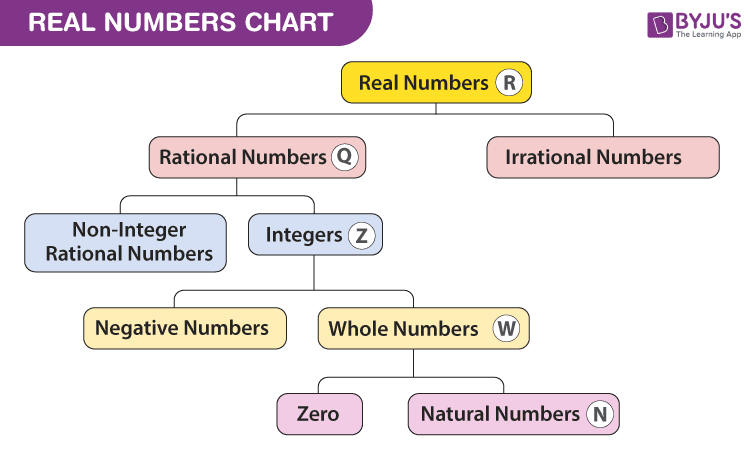To know more about real numbers, visit here.

Students can refer to the short notes and MCQ questions along with a separate solution pdf of this chapter for quick revision from the links below.

• Real Numbers Short Notes
• Real Numbers MCQ Practice Questions
• Real Numbers MCQ Practice Solutions
• ### Euclid’s Division Lemma

• Euclid’s Division Lemma states that given two integers a and b, there exists a unique pair of integers q and r such that a=b×q+r and 0r<b.
• This lemma is essentially equivalent to : dividend = divisor × quotient + remainder
• In other words, for a given pair of dividend and divisor, the quotient and remainder obtained are going to be unique.

#### For more information on Euclid’s Division Lemma, watch the below video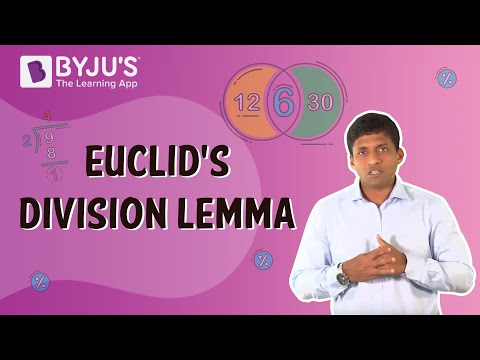To know more about Euclid’s Division Lemma, visit here.

### Euclid’s Division Algorithm

• Euclid’s Division Algorithm is a method used to find the H.C.F of two numbers, say a and b where a> b.
• We apply Euclid’s Division Lemma to find two integers q and r such that a=b×q+r and 0r<b.
• If r = 0, the H.C.F is b; else, we apply Euclid’s division Lemma to b (the divisor) and r (the remainder) to get another pair of quotient and remainder.
• The above method is repeated until a remainder of zero is obtained. The divisor in that step is the H.C.F. of the given set of numbers.

#### For more information on Euclid’s Division Algorithm, watch the below video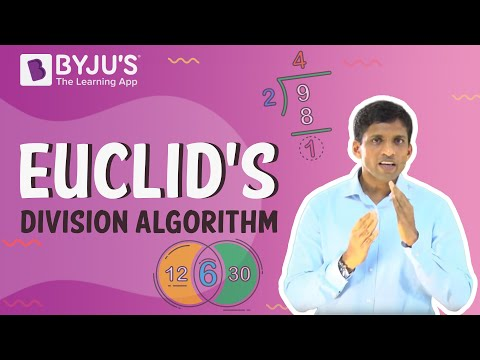## The Fundamental Theorem of Arithmetic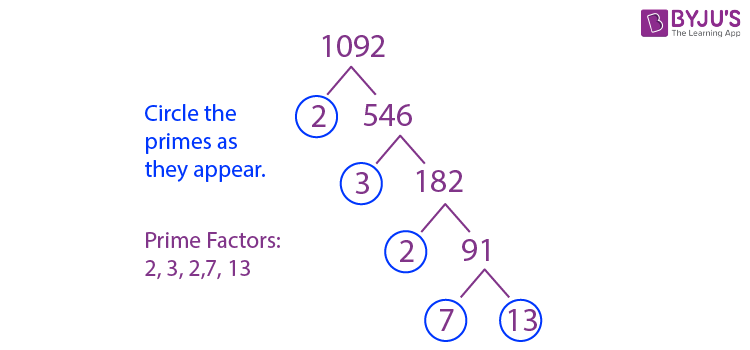### Prime Factorisation

• Prime Factorisation is the method of expressing a natural number as a product of prime numbers.
• Example: 36=2×2×3×3  is the prime factorisation of 36.

### Fundamental Theorem of Arithmetic

• The Fundamental Theorem of Arithmetic states that the prime factorisation for a given number is unique if the arrangement of the prime factors is ignored.
• Example: 36=2×2×3×3 OR, 36=2×3×2×3
• Therefore, 36 is represented as a product of prime factors (Two 2s and two 3s) ignoring the arrangement of the factors.

To know more about the Fundamental Theorem of Arithmetic, visit here.

### Method of Finding LCM

As we know, the smallest of the common multiples of two or more numbers is called the lowest common multiple (LCM).
Example: To find the Least Common Multiple  (L.C.M) of 36 and 56,

1.  36=2×2×3×3
56=2×2×2×7
2.  The common prime factors are 2×2
3.  The uncommon prime factors are  3×3 for 36 and 2×7 for 56.
4. LCM of 36 and 56 = 2×2×3×3×2×7  which is 504

To know more about LCM, visit here.

### Method of Finding HCF

We know that the greatest number that divides each of the given numbers without leaving any remainder is the highest common factor (HCF) of two or more given numbers.
H.C.F can be found using two methods – Prime factorisation and Euclid’s division algorithm.

• Prime Factorisation:
• Given two numbers, we express both of them as products of their respective prime factors. Then, we select the prime factors that are common to both the numbers
• Example – To find the H.C.F of 20 and 24
20=2×2×5   and   24=2×2×2×3
• The factor common to 20 and 24 is 2×2, which is 4, which in turn is the H.C.F of 20 and 24.
• Euclid’s Division Algorithm:
• It is the repeated use of Euclid’s division lemma to find the H.C.F of two numbers.
• Example: To find the HCF of 18 and 30
• The required HCF is 6.

To know more about HCF, visit here.

#### For more information on HCF and LCM, watch the below video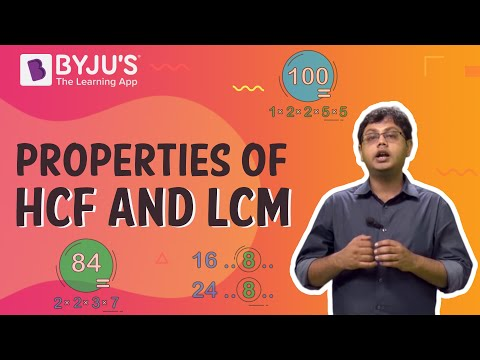To know more about the Properties of HCF and LCM, visit here.

### Product of Two Numbers = HCF X LCM of the Two Numbers

• For any two positive integers a and b,
a×b=H.C.F×L.C.M.
• Example – For 36 and 56, the H.C.F is 4 and the L.C.M is 504
36×56=2016
4×504=2016
Thus, 36×56=4×504
• Let us consider another example:
For 5 and 6, the H.C.F is 1 and the L.C.M is 30
5 × 6 = 30
1 × 30 =30
Thus, 5 × 6 = 1 × 30
• The above relationship, however, doesn’t hold true for 3 or more numbers

To know the Relationship between LCM and HCF, visit here.

### Applications of HCF & LCM in Real-World Problems

L.C.M can be used to find the points of common occurrence. For example,ringing of bells that ring with different frequencies, the time at which two persons running at different speeds meet, and so on.

#### For more information on Applications Of LCM, watch the below video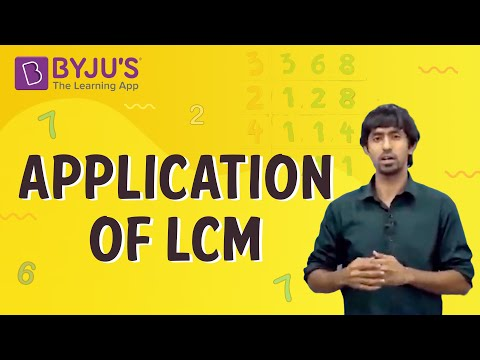## Revisiting Irrational Numbers

### Irrational Numbers

Any number that cannot be expressed in the form of p/q (where p and q are integers and q0.) is an irrational number. Examples √2,π, e and so on.

To know more about Irrational Numbers, visit here.

### Number theory: Interesting results

• If a number p (a prime number) divides a2, then p divides a. Example: 3 divides 62 i.e 36, which implies that 3 divides 6.
• The sum or difference of a rational and an irrational number is irrational
• The product and quotient of a non-zero rational and irrational number are irrational.
• √p is irrational when ‘p’ is a prime. For example, 7 is a prime number, and √7 is irrational. The above statement can be proved by the method of “Proof by contradiction”.

To know more about Number theory, visit here.

In the method of contradiction, to check whether a statement is TRUE
(i)  We assume that the given statement is TRUE.
(ii) We arrive at some result which contradicts our assumption, thereby proving the contrary.
Eg: Prove that √7 is irrational.
Assumption: √7  is rational.
Since it is rational √7 can be expressed as
√7 = a/b, where a and b are co-prime Integers, b 0.
On squaring, a2/b2=7
a2=7b2.
Hence, 7 divides a. Then, there exists a number c such that a=7c. Then, a2=49c2. Hence, 7b2=49c2 or b2=7c2.
Hence 7 divides b. Since 7 is a common factor for both a and b, it contradicts our assumption that a and b are coprime integers.
Hence, our initial assumption that √7  is rational is wrong. Therefore, √7 is irrational.

## Revisiting Rational Numbers and Their Decimal Expansions

### Rational Numbers

Rational numbers are numbers that can be written in the form p/q, where p and q are integers and q0.
Examples -1/2, 4/5, 1,0,3  and so on.

To know more about Rational Numbers, visit here.

### Terminating and Non-Terminating Decimals

Terminating decimals are decimals that end at a certain point. Example: 0.2, 2.56 and so on.
Non-terminating decimals are decimals where the digits after the decimal point don’t terminate. Example: 0.333333….., 0.13135235343…
Non-terminating decimals can be :
a) Recurring – a part of the decimal repeats indefinitely (0.142857142857….)
b) Non-recurring – no part of the decimal repeats indefinitely. Example: π=3.1415926535…

### Check if a given rational number is terminating or not

If a/b is a rational number, then its decimal expansion would terminate if both of the following conditions are satisfied :
a) The H.C.F of a and b is 1.
b) b can be expressed as a prime factorisation of 2 and 5 i.e b=2m×5n where either m or n, or both can = 0.
If the prime factorisation of b contains any number other  than 2 or 5, then the decimal expansion of that number will be recurring

Example:

1/40=0.025 is a terminating decimal,  as the H.C.F of 1 and 40 is 1, and the denominator (40) can be expressed as 23×51.

3/7=0.428571 is a recurring decimal as the H.C.F of 3 and 7 is 1 and the denominator (7) is equal to 71

## Real Numbers for Class 10 Solved Examples

Example 1:

Find the largest number that divides 70 and 125 leaving the remainder 5 and 8 respectively.

Solution:

First, subtract the remainder from the number.

(i.e) 70-5 = 65

125-8 = 117.

Thus, we need to find the largest number that divides 65 and 117 and leaves the remainder 0.

To find the largest number, take the HCF of 65 and 117.

Finding HCF of 65 and 117.

65 = 5×13

117 = 3×3×13.

Hence, HCF (65, 117) = 13.

Therefore, the largest number that divides 70 and 125 leaving the remainder 5 and 8 respectively is 13.

Example 2:

Find the LCM of 306 and 657, given that HCF (306, 657) = 9.

Solution:

Given that, HCF (306, 657) = 9.

We know that HCF × LCM = Product of Numbers

Hence, 9×LCM = 306×657

9×LCM = 201042

LCM = 201042/9

LCM = 22338.

Therefore, LCM of 306 and 657 is 22338.

Example 3:

Prove that 1/√2 is an irrational number.

Solution:

To prove 1/√2 is an irrational number.

Now, let us take the opposite assumption.

(i.e) Take 1/√2 is a rational number.

We know that rational numbers are the numbers that can be written in the form of p/q, where q is not equal to 0. (p and q are two co-prime numbers)

Hence, 1/√2 = p/q.

Now, simplify the above equation by multiplying √2 on both sides.

1 = (p√2)/q

q = p√2

Hence, we get q/p = √2.

Here, p and q are integers, and hence q/p is a rational number.

But, √2 is an irrational number.

Hence, our assumption is wrong.

Therefore, 1/√2 is an irrational number.

Hence, proved.

## Frequently Asked Questions on Class 10 Real Numbers

Q1

### What is Euclid’s division algorithm?

Euclid’s division algorithm is a method to find the Highest Common Factor of two given positive integers.
Q2

### What does real numbers for Class 10 explains?

In real numbers for Class 10, we will learn about Euclid’s division algorithm, the fundamental theorem of arithmetic, methods of finding LCM, HCF and the complete explanation of rational and irrational numbers.
Q3

### Is 10i a real number?

No, 10i is not a real number but an imaginary number.
Q4

### What does the Fundamental theory of Arithmetic explain?

The Fundamental Theorem of Arithmetic states that the prime factorisation for a given number is unique if the arrangement of the prime factors is ignored

Download BYJU’S – The Learning App and stay tuned with us to learn all Maths-related concepts easily by exploring more videos.

Test your Knowledge on Real Numbers for Class 10

1. Thanks its had helped very much

2. It is very easy to understand

3. awsome and clearly understandable

4. thankyou so much its very understandable

5. thank you very much
this info. was very useful
great job. THANK YOU

6. This notes is excellent

7. thanks it helped me so much

8. Thanks it was really helpful

9. thank you for this , helped in last minute revision.

10. Thank you so much for the notes.
It really helped me a lot

11. good it helped me

12. Thanks, it was very helpful to me 🤗

13. The notes are really good and helpful 👏

14. Thanks it helps me so much

15. Amazing and very useful!!

16. It’s amazing 😍😍 really helpful !! thanks

17. It really helped me in understanding this chapter 😊

18. Helpful for my understandings and notes are awesome 😁😁😄

19. Its really helpful thant byjus.
Its helps to understand, learn and revise within minimum time.

20. Thanks it is helpful so much

21. thank you sir for notes and test knowledge

22. Very useful and easy to understand the concept

23. Thankyou byju ‘s for this notes